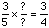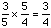Subject: Fractions Name: Whiteny Who is asking: Student Level: Middle Question: How many 3/5 are in 3/4? Hi Whitney, If you ask "How many 2's are in 8?" the answer is 4 since 2x4 = 8. Thus another way to ask your question is 3/5 x ?what? = 3/4 Since you expect the answer is a fraction you can rewrite thisBut you know how to multiply fractions so if you put a 5 in the numerator of the missing fraction and a 4 in the denominator, then the 5's cancel and you get 3/4. Thusand hence the number of 3/5's in 3/4 is 5/4. Cheers, Penny Go to Math Central